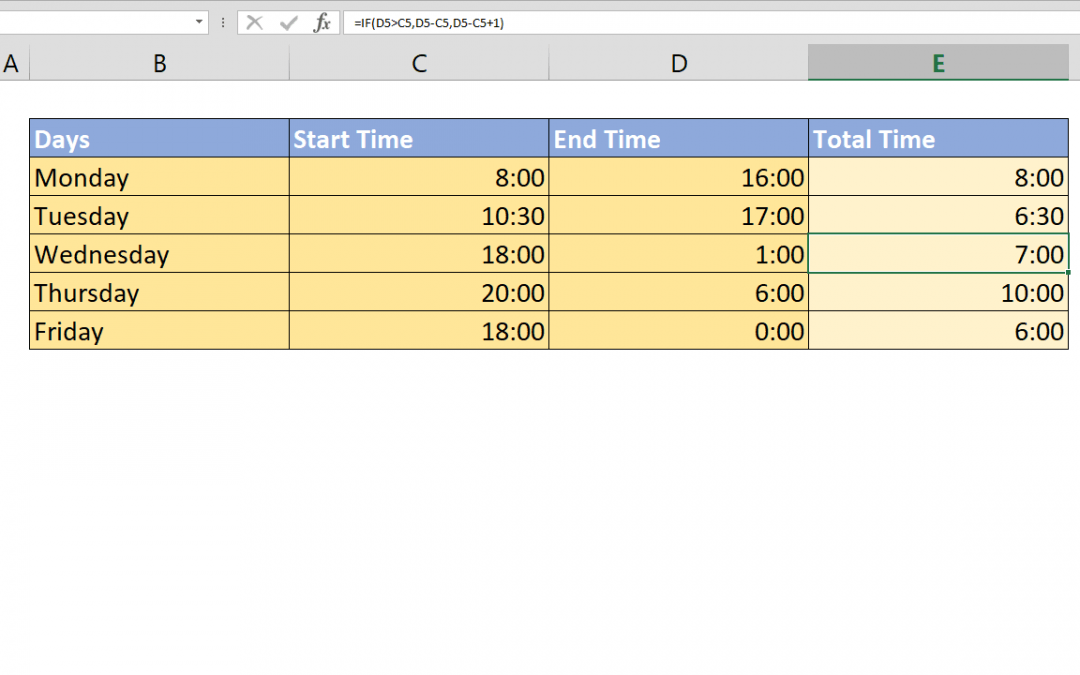Select Page## What week of the year is it and how to get its end date

Although Excel has a function to get week number of a date, WEEKNUM; there isn’t any to make reverse calculation. This method will help you answer the question – what week of the year is it, and give you the end date of each week. Syntax =MIN(DATE(current...## What week of the year is it and how to get its start date

Although Excel has a function to get week number of a date, WEEKNUM; there isn’t any to make reverse calculation. This method will help you answer the question – what week of the year is it, and give you the start date of each week. Syntax...## How to find unique values Excel

Managing and organizing data doesn’t have to be a daily routine. You can automated tasks like finding the outliers in your data. In this article, we’re going to show you how to find unique values Excel. Syntax { =IFERROR(   INDEX( data,     MATCH( 0,    ...## How to create a time calculator in Excel

When you need to calculate the difference between a time present day and the next day, calculations can get messed up and you will see lots of # characters. This article shows how to create a time calculator in Excel that can calculate shift time after midnight using...## How to find closest match

This article shows How to find closest match by using INDEX, MATCH, ABS and MIN functions. Excel’s array formula ability to evaluate formula for each cells in an array is key factor in this case. Syntax { =INDEX( return array,      MATCH(         MIN(ABS( search...